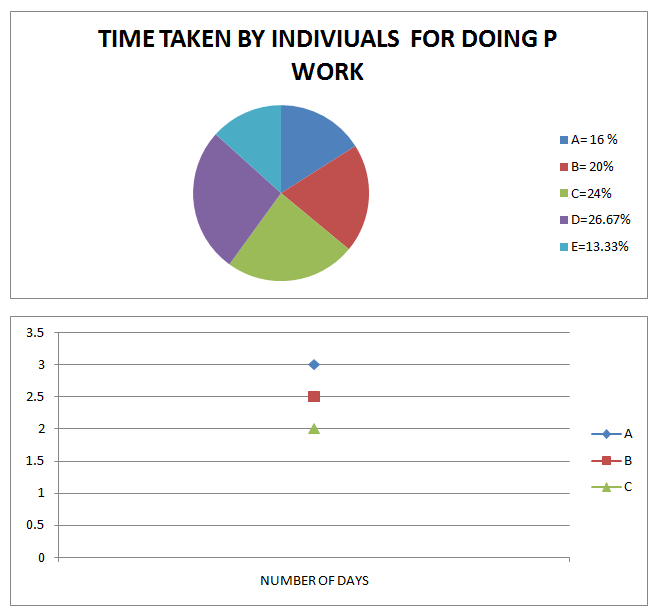# Quantitative Aptitude Questions (Data Interpretation and Application Sums) for IBPS Clerk Mains Day-29

## Quantitative Aptitude Questions (Data Interpretation and Application Sums) for IBPS Clerk Mains Day-29:

Dear Readers, IBPS is conducting Online Mains Examination for the recruitment of Clerical Cadre. Main Examination of IBPS Clerk was scheduled from 21st Jan 2018. To enrich your preparation here we have providing new series of Data Interpretation and Application Sums – Quantitative Aptitude Questions. Candidates those who are appearing in IBPS Clerk Main Exam can practice these Quantitative Aptitude Average questions daily and make your preparation effective.

### Click “Start Quiz” to attend these Questions and view Solutions

Directions(Q 1-5) The pie chart shows time taken by A, B, C, D and E individually do the work for P. Sum of time taken by A,B,C,D and E is 75 days. The Bar graph shows the number of days for A, B, C. Number of days for D and E is X and Y respectively.Q 1 If B and D alternatively does a work for 14 days and then work left is done by C. How many days does it take to complete the work?

1. 3.3 days
2. 2 days
3. 3 days
4. 4 days
5. None of these

Q2 If A do the work for 8 days and then C and E together do the remaining work. Then in how many days work will be completed?

1. 1 day
2. 3 1/7 days
3. 2 1/7 days
4. 4 days
5. None of these

Q3  If B, D and E do the job for 2  days respectively  and  remaining job is  done by A and C. A work  for  4 days then how many days does C take?

1. 1.2 days
2. 2.2 days
3. 3.2 days
4. 4.2 days
5. None of these

Q4 If ratio between x and y is 1:2 then find the number of days D work?

1. 2 days
2. 3 days
3. 4 days
4. 5 days
5. None of these

Q5.IF A,B and C works with an efficiency  of 133% ,150% and 83% on alternative days starting with A, then B and C respectively so how many days are required to complete work?

1. 4 days
2. 3 days
3. 8 days
4. 2 days
5. None of these

Q6 A, B, C went into the partnership with 5000, 6000, x respectively then after a quarter A withdraw 20% and B withdraw 16.667 %. And after 6 months, C increased 5.26% its investment. The profit ratio at the end of the year is in 170:210:156 then find the Value of x?

1. 3800
2. 3900
3. 3300
4. 3600
5. None of these

Q7.There is 60 cards. whose value is more

1. Probability of picking up a card whose digits, if interchanged, result in a number which is 27 less than the number picked up
2. Probability of picking up a card whose digits, if interchanged, result in a number which is 45 more than the number picked up
3. Probabality of picking up a card who is multiple of total conditions which can be drawn by sum of 2 dice thrown is 8.
1. 1>2>3
2. 3>2>1
3. 2>3>1
4. 3>1>2
5. None of these

Q8. Ankita borrowed money and returned it in 3 equal quarterly installments. What principal had she borrowed if the installment for each quarter is 2778.3 and rate of interest was 20%?

1. 7566
2. 6305
3. 12610
4. 8827
5. None of these

Q9. A vessel contains 4l milk and 2l of water if 20% contents are removed and 0.4l pure milk is added then x liters water is added to obtain reverse ratio. After those y liters of milk is added so as to make it reverse its ratio again. Find y?

1. 10.8l
2. 9l
3. 8.8l
4. 7.8l
5. None of these

Q10 Which value is more

1. Area of parallelogram with height and base is equal 8 and 10 respectively
2. Area of trapezium with parallel side is equal to 8 and 4 with height 8
3. Area of rhombus whose diagonals are 10 and 12 respectively
1. 1>2>3
2. B.3>2>1
3. 3>1>2
4. D.2>1>3
5. None of these